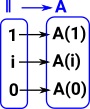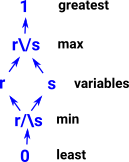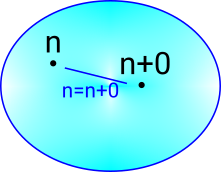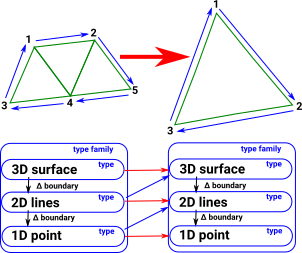# Maths - Cubical Type Theory

## Related Subjects

### Spaces As Types### The Formal Interval

HoTT uses an idea from topology, the interval.represents the unit interval [0,1]

not totally ordered like classic interval.is like a type but it is not a type. Instead a path is a primitive notion in HoTT.

### Paths

 PathAx y behaves like a function out of the interval p:-> A where: p 0 = x p 1 = y Althoughis not a proper type so we can't have a function into it for example.Transport turns path into equivalence.

### Comparison with Intentional Type Theory

In M-L intentional type theory a proposition is either proven or not, if it was not then it may, or may not, be true. Since proofs in HoTT are canonical then we have other possibilities, it may be true, false, neither or both, also it may be true in many ways. These values represent truth values of propositions which correspond to paths in a space. This situation with different truth values is related to the idea of topos in category theory.

ITT HoTT

Proposition

a=b

Path

I->A
may still use a=b

Proof of that proposition

Refl: a=a

λi -> x

### Free De Morgan Algebra

De Morgan algebra is a generalisation of boolean algebra without rule of excluded middle.

used for variables i...

with grammar

r,s ::= 0 | 1 | i | 1-r | r/\s | r\/s

where:

• 0 is least element 0:(1->)
• 1 is greatest element 1:(1->)
• r,s are variables
• r/\s is meet representing min /\:(->->)
• r\/s is join representing max \/:(->->)
• 1-r is an involution (its own inverse)
• where:
• 1-0=1
• 1-1=0
• 1-(r\/s) = (1-r)/\(1-s)
• 1-(r/\s) = (1-r)\/(1-s)
• (1-r):(->)#### Recap

So if we have 2 points 'n' and 'n+0' are they on a path/surface? This corresponds to the proposition 'n=n+0'. If we we using Boolean logic this proposition would be either true or false. In M-L type theory/constructive logic the proposition is either proven or its not, if its not then we can't say anything about it. Here the truth value is a free De Morgan algebra.### Fibrations

 A cubical complex might be thought of as a family of types where the cells are put into are hierarchy of types according to their dimension. These dimensions (and therefore types) are related by their boundary maps,### Presheaves

Presheaves allow us to build a more complex object out of simpler objects.

If C is a category of primitive objects then the presheaf is:

F: Cop -> Set

Objects of C Morphism in C
X
f: X -> Y
where:
F(X) is a set representing the ways X occurs inside F.

where:
F(f) : F(Y) -> F(X) is the function mapping each occurance y of Y in F to the corresponding suboccurrence x (included in y through f) of X in F

 see nlab siteCubical sets are presheaves

Sheaves are discussed on page here.

### Kan Composition## Implementation

Where I can, I have put links to Amazon for books that are relevant to the subject, click on the appropriate country flag to get more details of the book or to buy it from them.Computation and Reasoning - This book is about type theory. Although it is very technical it is aimed at computer scientists, so it has more discussion than a book aimed at pure mathematicians. It is especially useful for the coverage of dependant types.